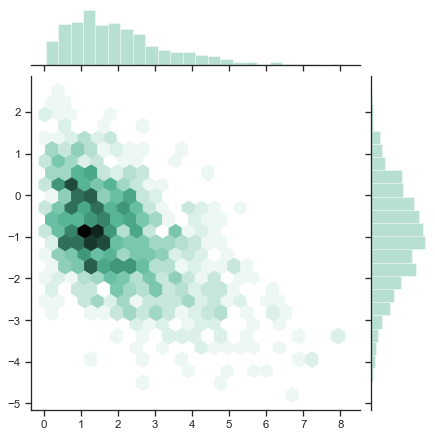# Hexbin plot with marginal distributions¶Python source code: `[download source: hexbin_marginals.py]`

```import numpy as np
import seaborn as sns
sns.set(style="ticks")

rs = np.random.RandomState(11)
x = rs.gamma(2, size=1000)
y = -.5 * x + rs.normal(size=1000)

sns.jointplot(x, y, kind="hex", color="#4CB391")
```